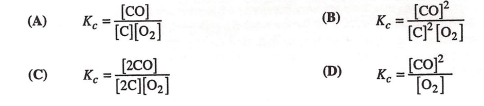# Problem: Consider this reaction.2 C(s) + O2 (g) ⇌ 2 CO (g)What is the equilibrium expression for this reaction?

###### FREE Expert Solution
86% (220 ratings)
###### Problem Details

Consider this reaction.

2 C(s) + O(g) ⇌ 2 CO (g)

What is the equilibrium expression for this reaction?What scientific concept do you need to know in order to solve this problem?

Our tutors have indicated that to solve this problem you will need to apply the Equilibrium Expressions concept. If you need more Equilibrium Expressions practice, you can also practice Equilibrium Expressions practice problems.

What is the difficulty of this problem?

Our tutors rated the difficulty ofConsider this reaction.2 C(s) + O2 (g) ⇌ 2 CO (g)What is the...as medium difficulty.

How long does this problem take to solve?

Our expert Chemistry tutor, Sabrina took 2 minutes and 58 seconds to solve this problem. You can follow their steps in the video explanation above.

What professor is this problem relevant for?

Based on our data, we think this problem is relevant for Professor Hoeger's class at UCSD.